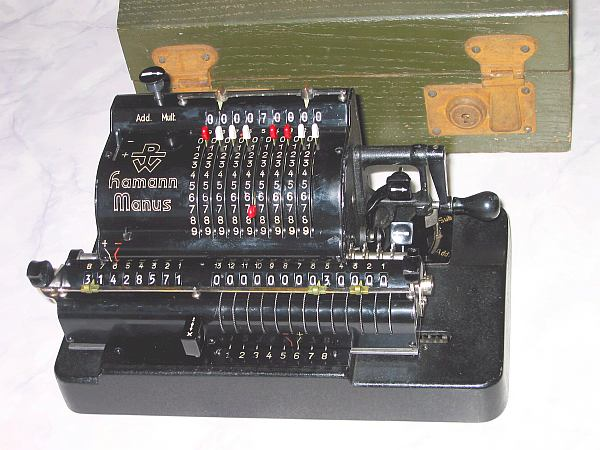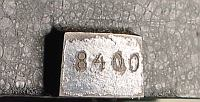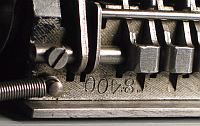previous <<==>> next

HAMANN-MANUS   » D «
(   Old Definition   =   MODEL   » E «   )*)   Have a look at the Restoration Procedure...
 ``` README the New Model Identification ... T E C H N I C A L D A T A of the Mechanical Calculator HAMANN-Manus *********************************************************************** ( New Identification ) Model » D « Serial Number: 8400 Dimensions: (ca.) Width = 10_1/4" / 26 cm Depth = 6 " / 15 cm Height = 6_1/4" / 16 cm Weight: (ca.) 13 lbs / 6 kg Mechanics: Switching-Latch-Wheel / Schalt-Klinke Functions: Add, Subtract, Multiply, Divide AUTOMATED DIVISION ! Registers: Input = 9 Decimals Counter = 8 Decimals Arithmetic = 13 Decimals Manufacturer: DTW ==>> DeTeWe Deutsche Telephon Werke AG Berlin / Germany 1935 H O W T O U S E the HAMANN-Manus » D « *********************************************** BASIC SETTINGS: =============== (A) Crank: ---------- In the idle position the crank is down and locked. To make one or more turns (clockwise only!) with the crank, pull out the handle to unlock. When finished the turn(s), let the handle snap in the lock. The locked down position only makes other functions (ex. clearing) accessible. REMARKS: Every started turn has to be finished completely! ******** Accidentally started turns are correctible somehow. The little lever under the name » HAMANN / MANUS « is only for "EMERGENCY" when the crank is blocked: Pulling the lever allows to crank backward. (B) Clear Input Unit: --------------------- Press the (->) knob on top to clear the input unit. When the knob is turned either to (Add <- ), than the input unit will be cleared after an operation, or turned to ( -> Mult), than the input unit will not be cleared after an operation. (C) Clear Counter & Arithmetic Unit in the Carriage: ---------------------------------------------------- Press-Down & Hold the [:/x] lever to shift the carriage to the far left position. This position is for clearing (and initial settings) only - No arithmetic operations are possible in this position! Only in this position it is possible to pre-set the arithmetic unit with the affiliated toothed wheels (13 ... 1). The right lever clears the arithmetic unit. With the left lever the counter is to clear also in all other positions of the carriage. *)  R E M A R K : It is the PURPOSE OF THE COGBAR IN THE BASE to set the carriage right for clearing arithmetic unit in all positions - BUT - It doesn't work for all possible conditions and positions ... (D) Set the Carriage in Start Position 1: ----------------------------------------- Pull the [:/x] front lever to [x] and push the [->] key once. (E) Set Counting Direction with the [+/-] Lever on Top to [+] ------------------------------------------------------------- In this case counter and arithmetic unit are working in the same direction; In case of [-] both are working in opposit direction. (F) Set the [Add/Sub] lever (under crank) to [Add]: --------------------------------------------------- This will set the carriage / arithmetic unit in [+] state; If set to [Sub], the carriage / arithmetic unit would be set in [-] state. ( The crank is turnable clockwise only! ) ADDITION & SUBTRACTION: ======================= Example: 123 + 45 - 6 = 162 ADD: Enter the first number (123) in the far right of the input unit. Make a (clockwise only!) turn with the crank to transfer input into arithmetic unit. The counting unit displays the figure 1. Enter the second number (45) into input unit. Turn the crank to add the number. The arithmetic unit displays the intermediate sum (168) and the counting unit displays the figure 2. SUBTRACT: Switch [Add/Sub] lever to [Sub]. Enter the third number (6) into input unit. Make a (clockwise only!) turn with the crank. The arithmetic unit displays the result (162) and the counting unit is decreased by 1. REMARK: NEGATIVE RESULTS are displayed in the arithmetic unit as the COMPLEMENT of the next higher 10, 100, 1000, ... Example: -12 = 99...9988 MULTIPLICATION: =============== Example: 123 x 45 = 5535 Clear registers and set (Add/Mult) knob to ( -> Mult). Enter the multiplicand (123) in the far right of the input unit. The multiplicator (45) has two digits, so the arithmetic unit is set to position 2 with a push on the [->] key . Make (clockwise only!) turns with the crank, until the first figure of the multiplicator (4) will appear in the 2nd position of the counter unit. Move the arithmetic unit to position 1 by pressing the [<-] key. Repeat making turns with the crank, until the second figure of the multiplicator (5) appears in the 1st position of the counter unit. The multiplication is done: The multiplicand (123) stays in the input, the multiplicator (45) is in the counter and the result (5535) is in the arithmetic unit. DIVISION: ========= Example: 22 : 7 = 3.1428571 Remainder 3 Set the (Add/Mult) knob to ( -> Mult); set the [Add/Sub] lever to [Sub]. In the "Basic Setting Position" (after clearing counter & arithmetic unit) set the dividend (22) in the far left of the arithmetic unit with the affiliated toothed wheels. Shift the carriage in position 8 and push the [:/x] lever to [:]. Set the divisor (7) into input unit above the dividend (22). Set Counting Direction Lever on Top to [-]. To devide make continuous (clockwise!) turns with the crank, until the division is finished. The algorithm (Subtraction until underflow, Add, Shift carriage, Continue...) is done automatically! The result (3.1428571) can be seen in the counter unit, and the remainder (3) is in the arithmetic unit. The divisor (7) is in the input unit, therefore an additional decimal can be estimated... ( 4, cause 4 x 7 = 28 ). Have a look at "Calculating Trickies" ... R E M A R K S : ======================================================================= The MANUS »D« came in green WOODEN BOX, original from the manufacturer, as the DTW BRAND proofs ...... and the CARRIAGE has the same SN = 8400 as its BASE. impressum: *********************************************************************** © C.HAMANN http://public.BHT-Berlin.de/hamann 12/15/08 ```